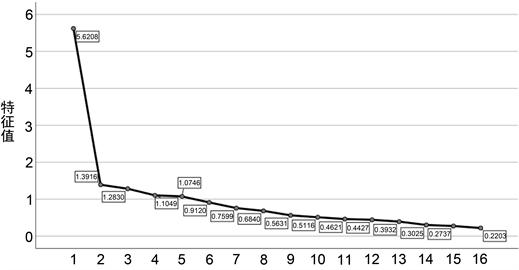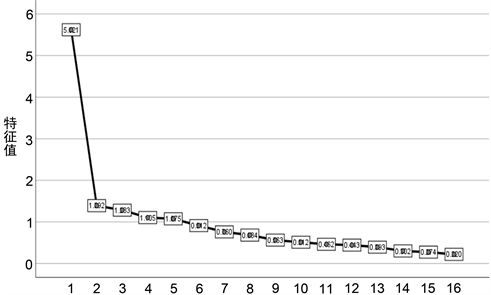﻿ 基于主成分分析的大学生网购行为影响因素分析

基于主成分分析的大学生网购行为影响因素分析Analysis of Influencing Factors of College Students’ Online Shopping Behavior Based on Principal Component Analysis

Abstract: With the popularization of computers and the development of the Internet, online shopping has gradually become a new way of shopping. College students are a special and huge online shopping group, and research on the influencing factors of their online shopping behavior can provide marketers with suggestions on product marketing strategies and product quality optimization, and also allow college students to understand their shopping preferences so as to reasonably plan their consumption. In this paper, principal component analysis is applied to analyze the behavioral factors affecting online shopping of male and female college students. It is concluded that the main factors that affect male online shopping are time, information, shop service, honesty factor and so on. The main factors affecting female online shopping include price, culture, concept, return and exchange, economic factors and so on. Finally, through data analysis, research conclusions are given and reasonable suggestions are given to businesses to guide the rational consumption of college students.

1. 引言

2. 研究设计

2.1. 主成分分析法

2.2. 影响因素体系Table 1. Factors affecting the online shopping behavior of college students

2.3. 抽样情况

2.4. 变量设计

3. 模型构建

3.1. 主成分的恰当性检验Table 2. KMO and Bartlett test

3.2. 主成分提取

3.2.1. 男生网购行为影响因素分析Figure 1. Lithotripsy map of influencing factors for male studentsTable 3. The eigenvalue and cumulative contribution of male factors

$\begin{array}{l}{F}_{1}=0.083{x}_{1}+0.098{x}_{2}+0.082{x}_{3}+0.103{x}_{4}+0.096{x}_{5}+0.107{x}_{6}+0.108{x}_{7}+0.107{x}_{8}\\ \text{\hspace{0.17em}}\text{\hspace{0.17em}}\text{\hspace{0.17em}}\text{\hspace{0.17em}}\text{\hspace{0.17em}}\text{\hspace{0.17em}}+0.108{x}_{9}+0.096{x}_{10}+0.098{x}_{11}+0.105{x}_{12}+0.087{x}_{13}+0.090{x}_{14}+0.097{x}_{15}+0.088{x}_{16};\end{array}$

$\begin{array}{l}{F}_{2}=0.363{x}_{1}+0.246{x}_{2}+0.398{x}_{3}+0.031{x}_{4}+0.133{x}_{5}-0153{x}_{6}-0.119{x}_{7}-0.153{x}_{8}\\ \text{\hspace{0.17em}}\text{\hspace{0.17em}}\text{\hspace{0.17em}}\text{\hspace{0.17em}}\text{\hspace{0.17em}}\text{\hspace{0.17em}}-0.087{x}_{9}-0.117{x}_{10}-0.013{x}_{11}+0.007{x}_{12}+0.150{x}_{13}-0.281{x}_{14}-0.245{x}_{15}+0.002{x}_{16};\end{array}$

$\begin{array}{l}{F}_{3}=0.141{x}_{1}-0.281{x}_{2}+0.031{x}_{3}+0.241{x}_{4}+0.050{x}_{5}+0.095{x}_{6}-0.068{x}_{7}-0.023{x}_{8}\\ \text{\hspace{0.17em}}\text{\hspace{0.17em}}\text{\hspace{0.17em}}\text{\hspace{0.17em}}\text{\hspace{0.17em}}\text{\hspace{0.17em}}-0.113{x}_{9}-0.408{x}_{10}-0.394{x}_{11}-0.030{x}_{12}+0.349{x}_{13}+0.266{x}_{14}+0.194{x}_{15}+0.067{x}_{16};\end{array}$

$\begin{array}{l}{F}_{4}=0.017{x}_{1}+0.034{x}_{2}+0.040{x}_{3}+0.048{x}_{4}-0.437{x}_{5}-0.324{x}_{6}-0.318{x}_{7}-0.131{x}_{8}\\ \text{\hspace{0.17em}}\text{\hspace{0.17em}}\text{\hspace{0.17em}}\text{\hspace{0.17em}}\text{\hspace{0.17em}}\text{\hspace{0.17em}}+0.041{x}_{9}-0.164{x}_{10}+0.285{x}_{11}+0.304{x}_{12}-0.040{x}_{13}+0.055{x}_{14}+0.306{x}_{15}+0.342{x}_{16};\end{array}$

3.2.2. 女生网购行为影响因素分析Figure 2. Lithotripsy map of influencing factors for female studentsTable 4. Eigenvalue and cumulative contribution of female influencing factors

$\begin{array}{l}{F}_{1}=0.107{x}_{1}+0.104{x}_{2}+0.115{x}_{3}+0.122{x}_{4}+0.076{x}_{5}+0.097{x}_{6}+0.107{x}_{7}+0.115{x}_{8}\\ \text{\hspace{0.17em}}\text{\hspace{0.17em}}\text{\hspace{0.17em}}\text{\hspace{0.17em}}\text{\hspace{0.17em}}\text{\hspace{0.17em}}+0.116{x}_{9}+0.115{x}_{10}+0.111{x}_{11}+0.097{x}_{12}+0.089{x}_{13}+0.114{x}_{14}+0.106{x}_{15}+0.083{x}_{16};\end{array}$

$\begin{array}{l}{F}_{2}=-0.172{x}_{1}-0.071{x}_{2}+0.111{x}_{3}-0.076{x}_{4}+0.255{x}_{5}-0.018{x}_{6}-0.101{x}_{7}+0.204{x}_{8}\\ \text{\hspace{0.17em}}\text{\hspace{0.17em}}\text{\hspace{0.17em}}\text{\hspace{0.17em}}\text{\hspace{0.17em}}\text{\hspace{0.17em}}\text{\hspace{0.17em}}-0.308{x}_{9}-0.362{x}_{10}-0.224{x}_{11}-0.094{x}_{12}+0.386{x}_{13}+0.338{x}_{14}-0.022{x}_{15}+0.119{x}_{16};\end{array}$

$\begin{array}{l}{F}_{3}=-0.044{x}_{1}-0.076{x}_{2}+0.174{x}_{3}-0.189{x}_{4}-0.291{x}_{5}-0.171{x}_{6}-0.250{x}_{7}+0.022{x}_{8}\\ \text{\hspace{0.17em}}\text{\hspace{0.17em}}\text{\hspace{0.17em}}\text{\hspace{0.17em}}\text{\hspace{0.17em}}\text{\hspace{0.17em}}+0.202{x}_{9}-0.045{x}_{10}-0.154{x}_{11}+0.147{x}_{12}+0.010{x}_{13}+0.154{x}_{14}+0.409{x}_{15}+0.500{x}_{16};\end{array}$

$\begin{array}{l}{F}_{4}=-0.085{x}_{1}+0.622{x}_{2}+0.371{x}_{3}-0.192{x}_{4}-0.018{x}_{5}-0.116{x}_{6}-0.224{x}_{7}-0.171{x}_{8}\\ \text{\hspace{0.17em}}\text{\hspace{0.17em}}\text{\hspace{0.17em}}\text{\hspace{0.17em}}\text{\hspace{0.17em}}\text{\hspace{0.17em}}+0.058{x}_{9}+0.105{x}_{10}-0.040{x}_{11}-0.375{x}_{12}+0.065{x}_{13}-0.019{x}_{14}-0.179{x}_{15}+0.223{x}_{16};\end{array}$

$\begin{array}{l}{F}_{5}=-0.173{x}_{1}-0.047{x}_{2}+0.055{x}_{3}-0.109{x}_{4}+0.106{x}_{5}-0.403{x}_{6}-0.182{x}_{7}-0.280{x}_{8}\\ \text{\hspace{0.17em}}\text{\hspace{0.17em}}\text{\hspace{0.17em}}\text{\hspace{0.17em}}\text{\hspace{0.17em}}\text{\hspace{0.17em}}+0.116{x}_{9}+0.012{x}_{10}+0.348{x}_{11}+0.490{x}_{12}+0.346{x}_{13}+0.161{x}_{14}-0.235{x}_{15}-0.147{x}_{16};\end{array}$

4. 结果分析和建议

4.1. 男生结果分析

F1，F2，F3，F4四个主成分分别从时间，信息，店铺服务，诚信，这几个角度来反应大学生网购影响情况。

$F=0.413{F}_{1}+0.102{F}_{2}+0.083{F}_{3}+0.075{F}_{4};$

4.2. 女生结果分析

F1，F2，F3，F4，F5五个主成分分别从价格，文化，观念，退换货，经济这几个角度来反应大学生网购影响情况。

$F=0.351{F}_{1}+0.087{F}_{2}+0.080{F}_{3}+0.069{F}_{4}+0.067{F}_{5};$

5. 结论

 Varki, S. and Colgate, M. (2001) The Role of Price Perceptions in an Integrated Model of Behavioral Intentions. Journal of Service Research, 3, 232-240.
https://doi.org/10.1177/109467050133004

 Corritore, C.L., Kracher, B. and Wiedenbeck, S. (2003) Online Trust: Concepts, Evolving Themes, A Model. International Journal of Human-Computer Studies, 58, 737-758.
https://doi.org/10.1016/S1071-5819(03)00041-7

 Pratminingsih, S.A., Linpuringtyas, C. and Rimenta, T. (2013) Factors Influencing Customer Loyalty toward Online Shopping. International Journal of Trade, Economics and Finance, 4, 104-110.
httpss://doi.org/10.7763/IJTEF.2013.V4.268

 吴景峰, 常亚平, 侯德林. 网络商店形象对情感反应和在线冲动性购买意愿的影响[J]. 商业经济与管理. 2012(8): 35-44.

 姜参, 赵宏霞, 孟雷. B2C网络购物在线互动与消费者冲动性购买行为研究[J]. 经济问题探索, 2014(5): 64-73.

 高晓倩, 王丽娟, 李蕊. 消费者心流体验对网络强迫性购买倾向的影响[J]. 商业研究, 2009, 12(2): 132-140.

 李广来. 大学生网购行为影响因素及企业营销策略研究[D]: [硕士学位论文]. 哈尔滨: 黑龙江大学, 2018.

Top